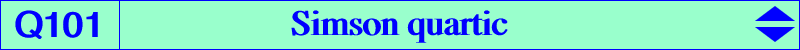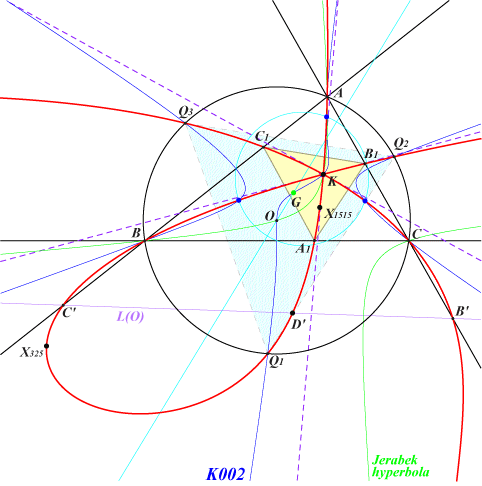too complicated to be written here. Click on the link to download a text file.X(6), X(325), X(1515), X(2574), X(2575), X(8779), X(10564), X(32112), X(41389), X(41390), X(52038), X(57466), X(57467), X(57468), X(57469), X(57470) Q1, Q2, Q3 vertices of the Thomson triangle A', B', C' : traces of the trilinear polar L(O) of O D' = X(3) x X(1503) on L(O) details and other points described belowConsider a variable point M on the circumcircle (O) and let S(M), L(M) be the Simson line, the trilinear polar of M respectively. These lines meet at P and when M traverses (O) the locus of P is the Simson quartic Q101. Q101 is an unicursal quartic since the Lemoine point K is a triple point on the curve obtained when M is one of the points on (O) whose Simson line passes through K. These points are the intersections (other than X(74)) of (O) and the image of the Jerabek hyperbola under the translation that maps H onto K (construction due to Jean-Pierre Ehrmann). Recall that L(M) always contains K. Q101 meets the trilinear polar L(O) of O at four points : A', B', C' obtained when M is a vertex of the circumcevian triangle of O and D', the barycentric product of O and X(1503), obtained when M = X(112). See a generalization below. A', B', C' lie on the sidelines of ABC therefore there are three other points A1, B1, C1 of Q101 on these same sidelines. They are obtained when M is the antipode on (O) of one vertex of the circumcevian triangle of G. Q101 meets (O) at A, B, C and the vertices Q1, Q2, Q3 of the Thomson triangle obtained when M is a vertex of the reflection of this same triangle about O. Recall that Q1, Q2, Q3 lie on the rectangular hyperbola passing through G, O, K, X(110), etc, whose asymptotes are parallel to those of the Jerabek hyperbola. Q101 meets the line at infinity at four points : two are always real (being the infinite points of the Jerabek hyperbola) and two are always imaginary conjugates (being the infinite points of the circum-ellipse with perspector X(574)). Q101 meets the Thomson cubic K002 at twelve points namely A, B, C, K (thrice), Q1, Q2, Q3 and three other (blue) points on the nine point circle. Collinear points on Q101 Let M be a point on the Jerabek hyperbola (J) and let (O') be the image of the circumcircle (O) under the translation T that maps M onto H. (J) and (O') intersect at four points : one of them is T(X74) and the three remaining (not necessarily all real) points are denoted T1, T2, T3. Let M1, M2, M3 be their reflections about the complement N of M. These points lie on (O) and on pK(X6, aM) where aM is the anticomplement of M. The Simson line of Mi is the line TjTk meeting the trilinear polar L(Mi) at Pi which lies on Q101. Furthermore, these points P1, P2, P3 are collinear on the line l(M) which meets Q101 at a fourth point P0. When M traverses (J), the locus of the trilinear pole of l(M) is the nodal cubic K657. If M = H, we find l(M) = L(O) as above and pK(X6, aM) is K004. If M = O, l(M) is the de Longchamps axis and pK(X6, aM) is K006.